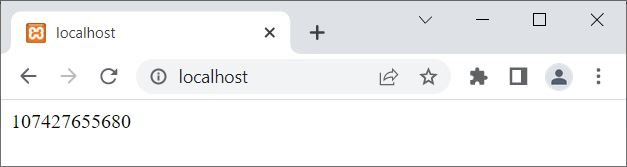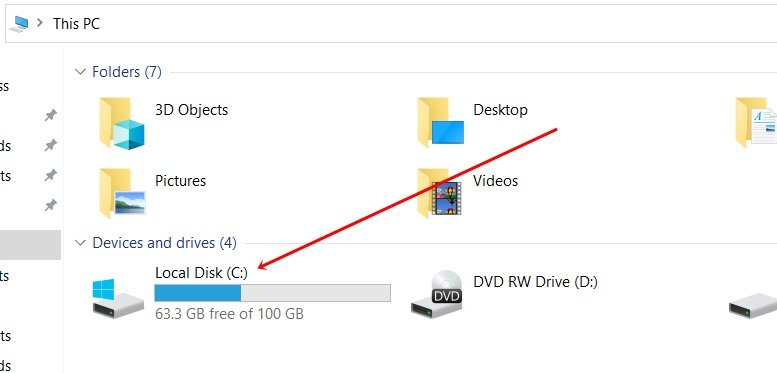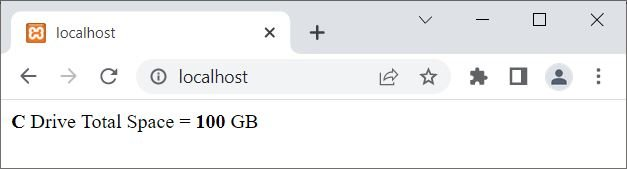# PHP disk_total_space(): Find total disk space

The PHP disk_total_space() function is used when we need to find the total space available on a specified disk or filesystem. The space returned using this function will be in bytes by default. For example:

```<?php
\$space = disk_total_space("C:");
echo \$space;
?>```

The output of the above PHP example on the disk_total_space() function is:That is, 107427655680 bytes are the total space available on my C drive. Here is a snapshot of the C drive of my computer system:## Find Amount of Total Disk Space in GB using PHP

To modify the above program to print the total space available on the C drive in GB, then use the following example:

```<?php
\$spaceBytes = disk_total_space("C:");

\$spaceKb = \$spaceBytes/1024;
\$spaceMb = \$spaceKb/1024;
\$spaceGb = \$spaceMb/1024;

echo "<p>Total Space available in <b>C</b> Drive is <b>\$spaceGb</b> GB</p>";
?>```

Now the output should be:To remove all digits after decimals, use (int) before \$spaceGb. For example:

```<?php
\$spaceBytes = disk_total_space("C:");

\$spaceGb = \$spaceBytes/1024/1024/1024;
\$spaceGb = (int)\$spaceGb;

echo "<p><b>C</b> Drive Total Space = <b>\$spaceGb</b> GB</p>";
?>```

Now the output should be:## PHP disk_total_space() Syntax

The syntax of the disk_total_space() function in PHP is:

`disk_total_space(x)`

The x parameter is required and refers to the filesystem or disk whose total space we need to see.

### Advantages of the disk_total_space() function in PHP

• Determining the overall size of a disk or partition is simple and can be done with a single line of code.
• It is fast because it only needs to determine the total size of the disk or partition.
• It's great for large disks or partitions because it doesn't hog a lot of memory.
• The total size of the disk or partition, including any reserved or hidden sectors, is accurately represented.

### Disadvantages of the disk_total_space() function in PHP

• This function does not reveal anything about the contents of the disk or partition other than its overall size.
• If the disk or partition being checked is under the user's control, then this function may pose a security risk. This might give nefarious users access to delicate server disk or partition information.
• The behavior of this function may vary across platforms and operating systems, which may have an impact on how accurately the total size is measured.
• If the disk or partition cannot be accessed or if there are other problems with the disk or partition, this function does not provide detailed error messages.

PHP Online Test

« Previous Tutorial Next Tutorial »